# FirstOrder Logic Review 1 Firstorder logic Firstorder logic

• Slides: 26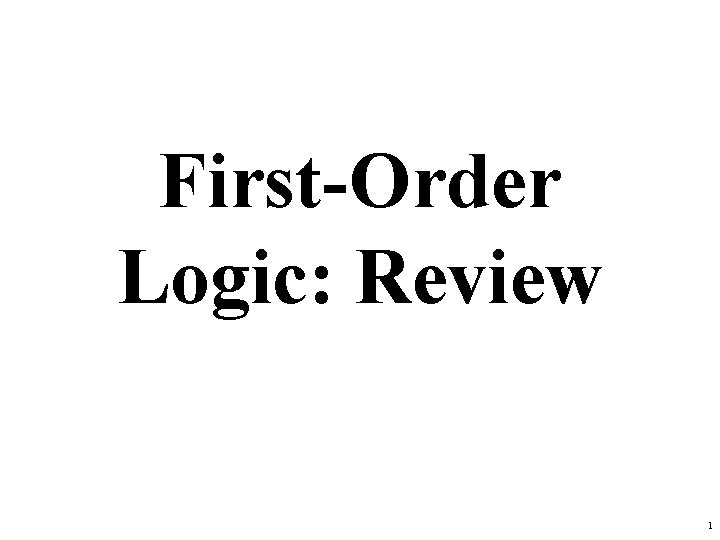First-Order Logic: Review 1First-order logic • First-order logic (FOL) models the world in terms of – Objects, which are things with individual identities – Properties of objects that distinguish them from others – Relations that hold among sets of objects – Functions, which are a subset of relations where there is only one “value” for any given “input” • Examples: – Objects: Students, lectures, companies, cars. . . – Relations: Brother-of, bigger-than, outside, part-of, hascolor, occurs-after, owns, visits, precedes, . . . – Properties: blue, oval, even, large, . . . – Functions: father-of, best-friend, second-half, more-than. . .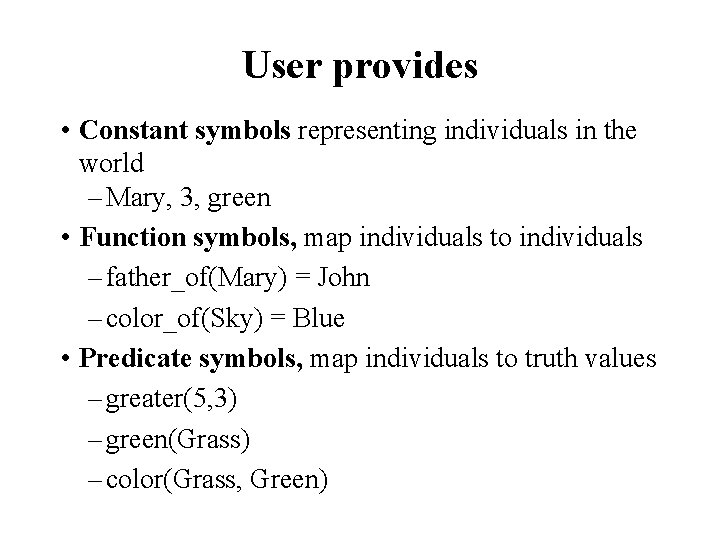User provides • Constant symbols representing individuals in the world – Mary, 3, green • Function symbols, map individuals to individuals – father_of(Mary) = John – color_of(Sky) = Blue • Predicate symbols, map individuals to truth values – greater(5, 3) – green(Grass) – color(Grass, Green)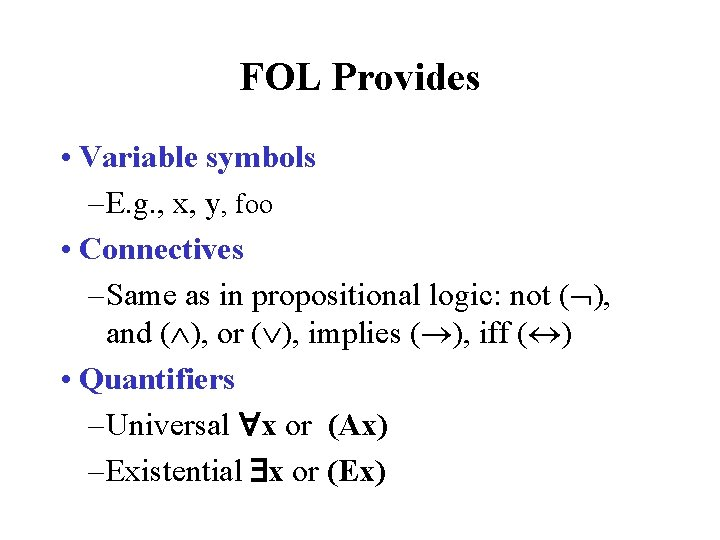FOL Provides • Variable symbols – E. g. , x, y, foo • Connectives – Same as in propositional logic: not ( ), and ( ), or ( ), implies ( ), iff ( ) • Quantifiers – Universal x or (Ax) – Existential x or (Ex)Sentences: built from terms and atoms • A term (denoting a real-world individual) is a constant symbol, variable symbol, or n-place function of n terms. • Examples of terms: – Constants: john, umbc – Variables: x, y, z – Functions: mother_of(john), phone(mother(x)) • Ground terms have no variables in them – A term with no variables is a ground term, i. e. , john, father_of(john))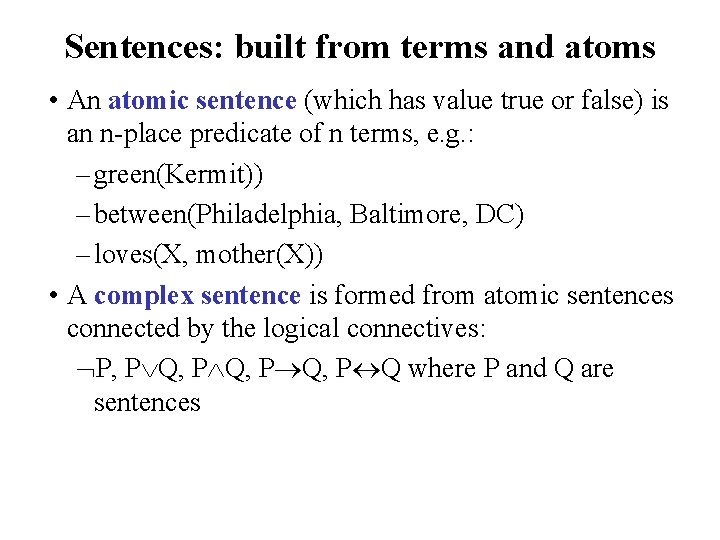Sentences: built from terms and atoms • An atomic sentence (which has value true or false) is an n-place predicate of n terms, e. g. : – green(Kermit)) – between(Philadelphia, Baltimore, DC) – loves(X, mother(X)) • A complex sentence is formed from atomic sentences connected by the logical connectives: P, P Q, P Q where P and Q are sentences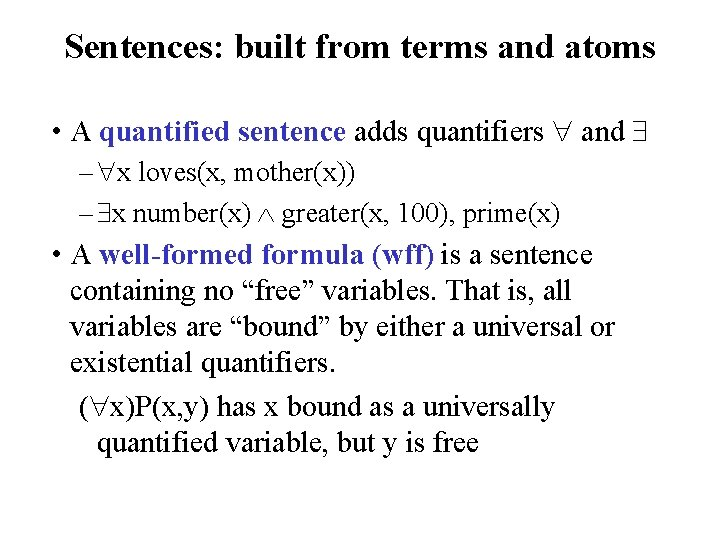Sentences: built from terms and atoms • A quantified sentence adds quantifiers and – x loves(x, mother(x)) – x number(x) greater(x, 100), prime(x) • A well-formed formula (wff) is a sentence containing no “free” variables. That is, all variables are “bound” by either a universal or existential quantifiers. ( x)P(x, y) has x bound as a universally quantified variable, but y is free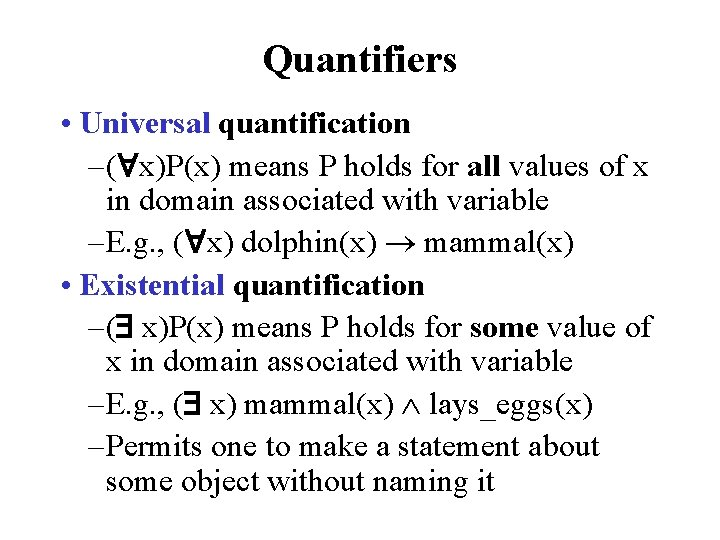Quantifiers • Universal quantification – ( x)P(x) means P holds for all values of x in domain associated with variable – E. g. , ( x) dolphin(x) mammal(x) • Existential quantification – ( x)P(x) means P holds for some value of x in domain associated with variable – E. g. , ( x) mammal(x) lays_eggs(x) – Permits one to make a statement about some object without naming it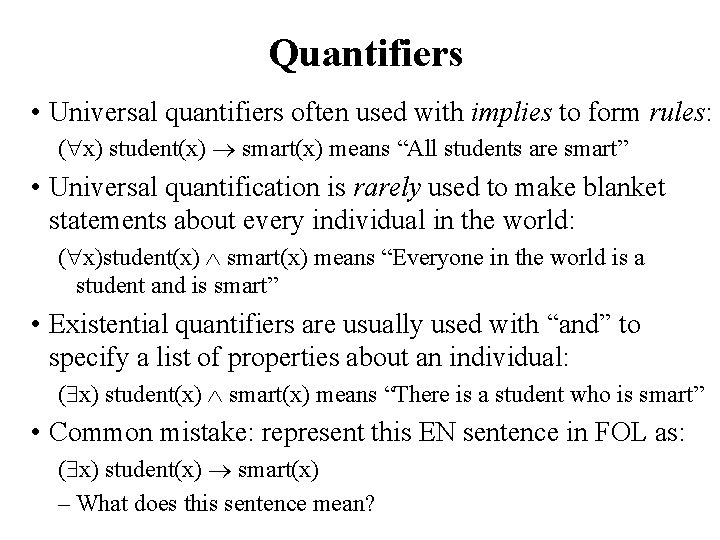Quantifiers • Universal quantifiers often used with implies to form rules: ( x) student(x) smart(x) means “All students are smart” • Universal quantification is rarely used to make blanket statements about every individual in the world: ( x)student(x) smart(x) means “Everyone in the world is a student and is smart” • Existential quantifiers are usually used with “and” to specify a list of properties about an individual: ( x) student(x) smart(x) means “There is a student who is smart” • Common mistake: represent this EN sentence in FOL as: ( x) student(x) smart(x) – What does this sentence mean?Quantifier Scope • FOL sentences have structure, like programs • In particular, the variables in a sentence have a scope • For example, suppose we want to say – “everyone who is alive loves someone” – ( x) alive(x) ( y) loves(x, y) • Here’s how we scope the variables ( x) alive(x) ( y) loves(x, y) Scope of x Scope of y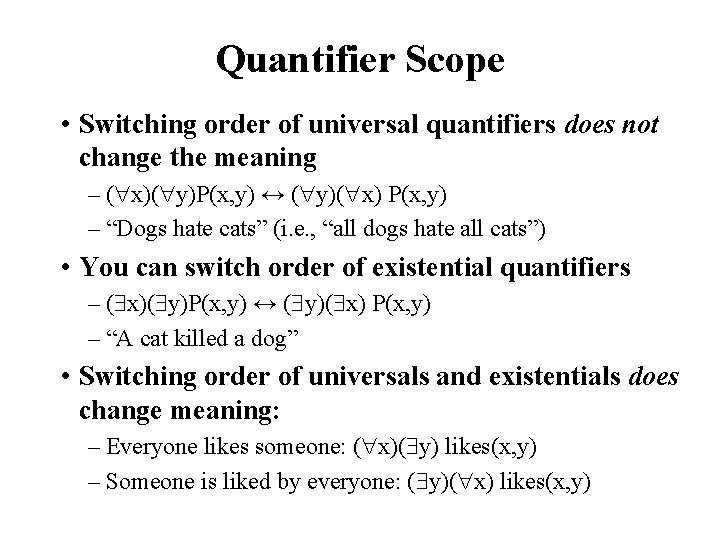Quantifier Scope • Switching order of universal quantifiers does not change the meaning – ( x)( y)P(x, y) ↔ ( y)( x) P(x, y) – “Dogs hate cats” (i. e. , “all dogs hate all cats”) • You can switch order of existential quantifiers – ( x)( y)P(x, y) ↔ ( y)( x) P(x, y) – “A cat killed a dog” • Switching order of universals and existentials does change meaning: – Everyone likes someone: ( x)( y) likes(x, y) – Someone is liked by everyone: ( y)( x) likes(x, y)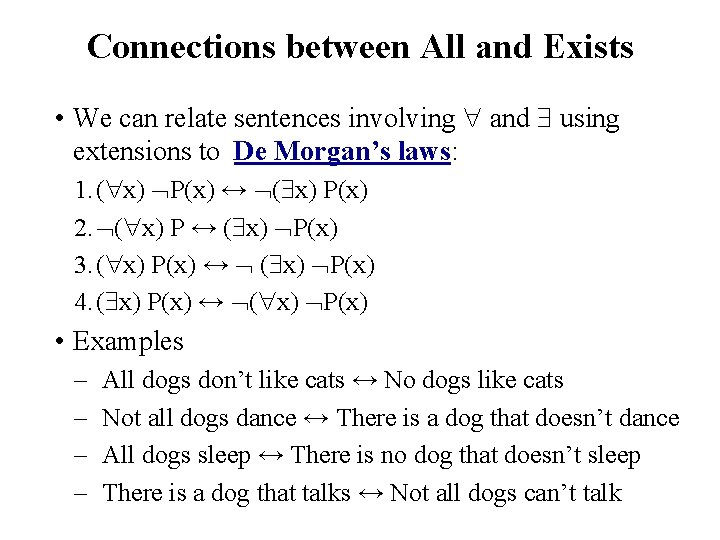Connections between All and Exists • We can relate sentences involving and using extensions to De Morgan’s laws: 1. ( x) P(x) ↔ ( x) P(x) 2. ( x) P ↔ ( x) P(x) 3. ( x) P(x) ↔ ( x) P(x) 4. ( x) P(x) ↔ ( x) P(x) • Examples – – All dogs don’t like cats ↔ No dogs like cats Not all dogs dance ↔ There is a dog that doesn’t dance All dogs sleep ↔ There is no dog that doesn’t sleep There is a dog that talks ↔ Not all dogs can’t talk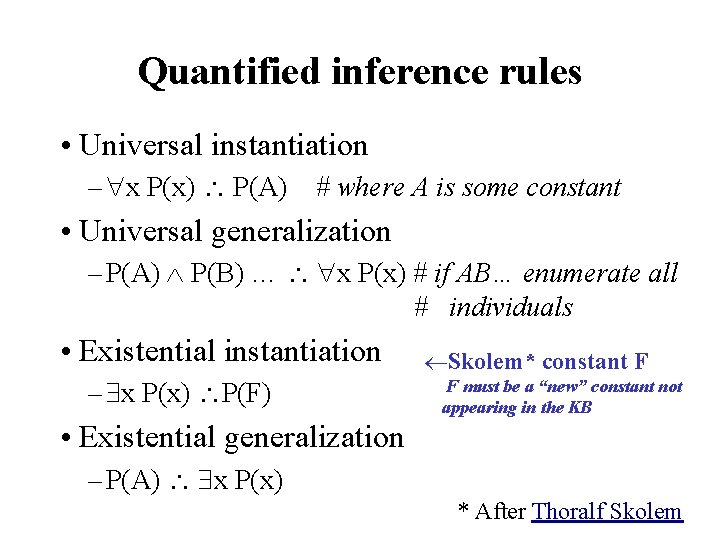Quantified inference rules • Universal instantiation – x P(x) P(A) # where A is some constant • Universal generalization – P(A) P(B) … x P(x) # if AB… enumerate all # individuals • Existential instantiation – x P(x) P(F) Skolem* constant F F must be a “new” constant not appearing in the KB • Existential generalization – P(A) x P(x) * After Thoralf Skolem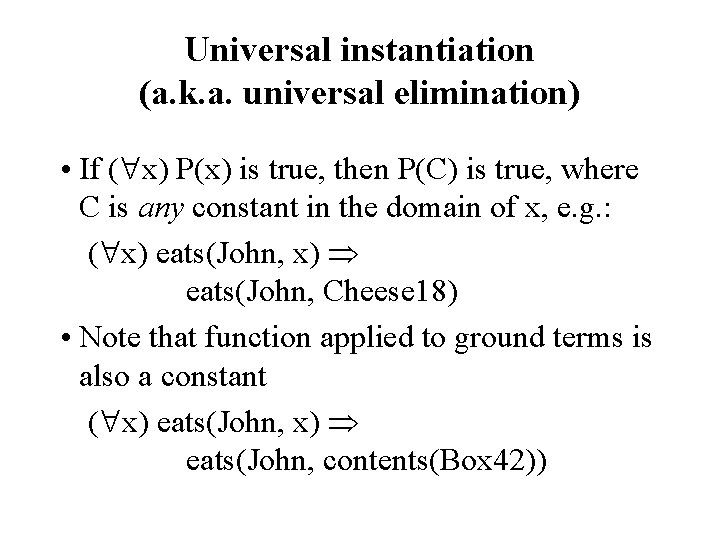Universal instantiation (a. k. a. universal elimination) • If ( x) P(x) is true, then P(C) is true, where C is any constant in the domain of x, e. g. : ( x) eats(John, x) eats(John, Cheese 18) • Note that function applied to ground terms is also a constant ( x) eats(John, x) eats(John, contents(Box 42))Existential instantiation (a. k. a. existential elimination) • From ( x) P(x) infer P(c), e. g. : – ( x) eats(Mikey, x) eats(Mikey, Stuff 345) • The variable is replaced by a brand-new constant not occurring in this or any sentence in the KB • Also known as skolemization; constant is a skolem constant • We don’t want to accidentally draw other inferences about it by introducing the constant • Can use this to reason about unknown objects, rather than constantly manipulating existential quantifiersExistential generalization (a. k. a. existential introduction) • If P(c) is true, then ( x) P(x) is inferred, e. g. : Eats(Mickey, Cheese 18) ( x) eats(Mickey, x) • All instances of the given constant symbol are replaced by the new variable symbol • Note that the variable symbol cannot already exist anywhere in the expression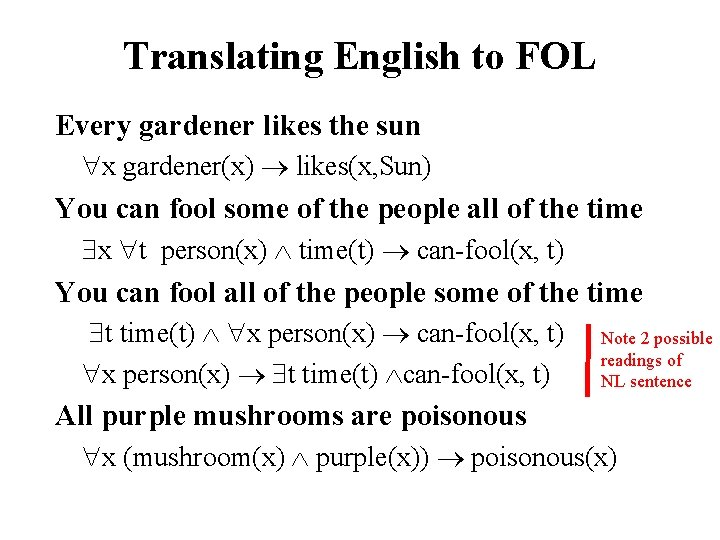Translating English to FOL Every gardener likes the sun x gardener(x) likes(x, Sun) You can fool some of the people all of the time x t person(x) time(t) can-fool(x, t) You can fool all of the people some of the time t time(t) x person(x) can-fool(x, t) x person(x) t time(t) can-fool(x, t) Note 2 possible readings of NL sentence All purple mushrooms are poisonous x (mushroom(x) purple(x)) poisonous(x)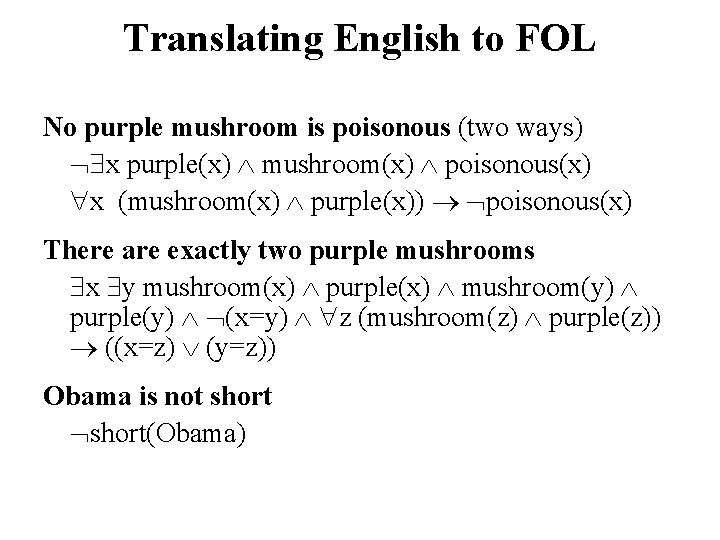Translating English to FOL No purple mushroom is poisonous (two ways) x purple(x) mushroom(x) poisonous(x) x (mushroom(x) purple(x)) poisonous(x) There are exactly two purple mushrooms x y mushroom(x) purple(x) mushroom(y) purple(y) (x=y) z (mushroom(z) purple(z)) ((x=z) (y=z)) Obama is not short(Obama)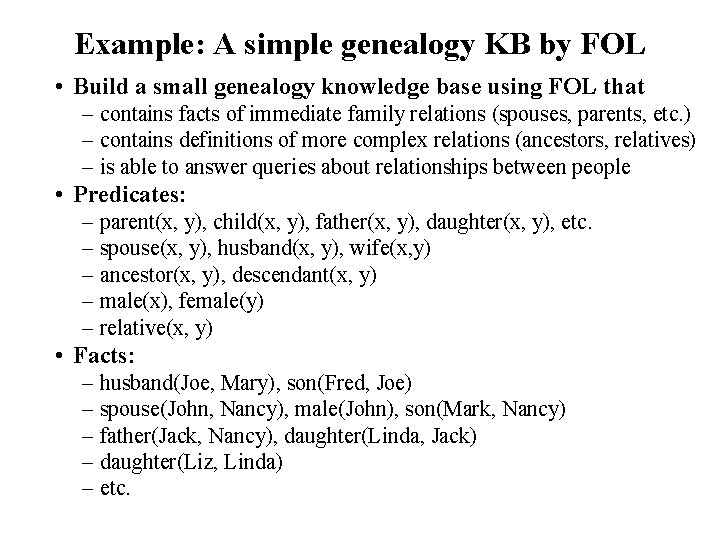Example: A simple genealogy KB by FOL • Build a small genealogy knowledge base using FOL that – contains facts of immediate family relations (spouses, parents, etc. ) – contains definitions of more complex relations (ancestors, relatives) – is able to answer queries about relationships between people • Predicates: – parent(x, y), child(x, y), father(x, y), daughter(x, y), etc. – spouse(x, y), husband(x, y), wife(x, y) – ancestor(x, y), descendant(x, y) – male(x), female(y) – relative(x, y) • Facts: – husband(Joe, Mary), son(Fred, Joe) – spouse(John, Nancy), male(John), son(Mark, Nancy) – father(Jack, Nancy), daughter(Linda, Jack) – daughter(Liz, Linda) – etc.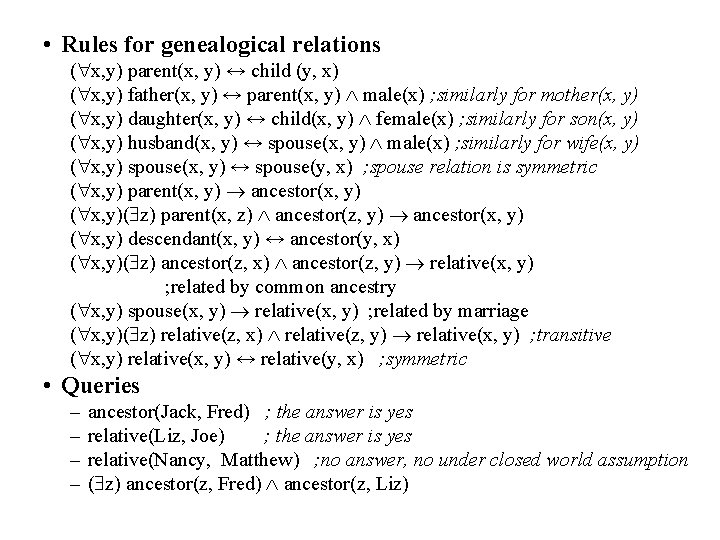• Rules for genealogical relations ( x, y) parent(x, y) ↔ child (y, x) ( x, y) father(x, y) ↔ parent(x, y) male(x) ; similarly for mother(x, y) ( x, y) daughter(x, y) ↔ child(x, y) female(x) ; similarly for son(x, y) ( x, y) husband(x, y) ↔ spouse(x, y) male(x) ; similarly for wife(x, y) ( x, y) spouse(x, y) ↔ spouse(y, x) ; spouse relation is symmetric ( x, y) parent(x, y) ancestor(x, y) ( x, y)( z) parent(x, z) ancestor(z, y) ancestor(x, y) ( x, y) descendant(x, y) ↔ ancestor(y, x) ( x, y)( z) ancestor(z, x) ancestor(z, y) relative(x, y) ; related by common ancestry ( x, y) spouse(x, y) relative(x, y) ; related by marriage ( x, y)( z) relative(z, x) relative(z, y) relative(x, y) ; transitive ( x, y) relative(x, y) ↔ relative(y, x) ; symmetric • Queries – – ancestor(Jack, Fred) ; the answer is yes relative(Liz, Joe) ; the answer is yes relative(Nancy, Matthew) ; no answer, no under closed world assumption ( z) ancestor(z, Fred) ancestor(z, Liz)Axioms, definitions and theorems • Axioms are facts and rules that attempt to capture all of the (important) facts and concepts about a domain; axioms can be used to prove theorems – Mathematicians don’t want any unnecessary (dependent) axioms, i. e. ones that can be derived from other axioms – Dependent axioms can make reasoning faster, however – Choosing a good set of axioms for a domain is a design problem • A definition of a predicate is of the form “p(X) ↔ …” and can be decomposed into two parts – Necessary description: “p(x) …” – Sufficient description “p(x) …” – Some concepts don’t have complete definitions (e. g. , person(x))More on definitions Example: define father(x, y) by parent(x, y) and male(x) • parent(x, y) is a necessary (but not sufficient) description of father(x, y) parent(x, y) • parent(x, y) ^ male(x) ^ age(x, 35) is a sufficient (but not necessary) description of father(x, y): father(x, y) parent(x, y) ^ male(x) ^ age(x, 35) • parent(x, y) ^ male(x) is a necessary and sufficient description of father(x, y) parent(x, y) ^ male(x) ↔ father(x, y)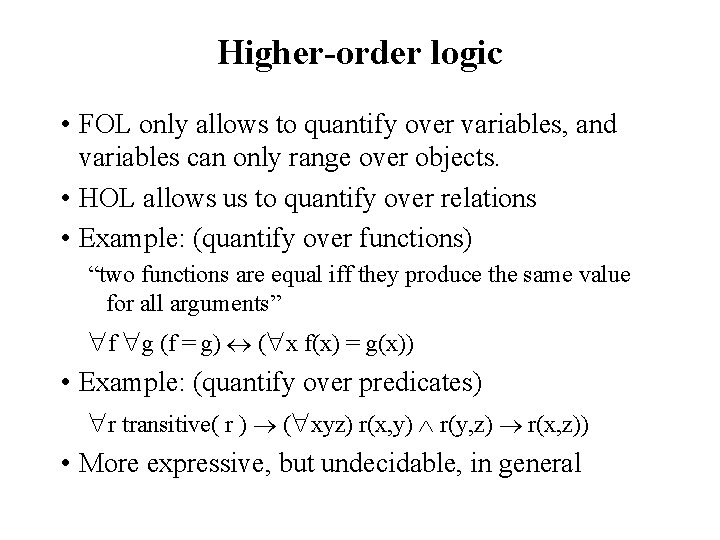Higher-order logic • FOL only allows to quantify over variables, and variables can only range over objects. • HOL allows us to quantify over relations • Example: (quantify over functions) “two functions are equal iff they produce the same value for all arguments” f g (f = g) ( x f(x) = g(x)) • Example: (quantify over predicates) r transitive( r ) ( xyz) r(x, y) r(y, z) r(x, z)) • More expressive, but undecidable, in general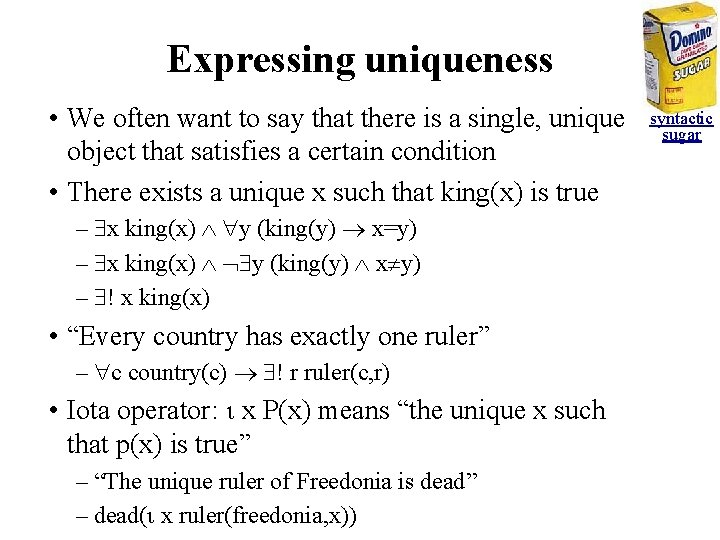Expressing uniqueness • We often want to say that there is a single, unique object that satisfies a certain condition • There exists a unique x such that king(x) is true – x king(x) y (king(y) x=y) – x king(x) y (king(y) x y) – ! x king(x) • “Every country has exactly one ruler” – c country(c) ! r ruler(c, r) • Iota operator: x P(x) means “the unique x such that p(x) is true” – “The unique ruler of Freedonia is dead” – dead( x ruler(freedonia, x)) syntactic sugar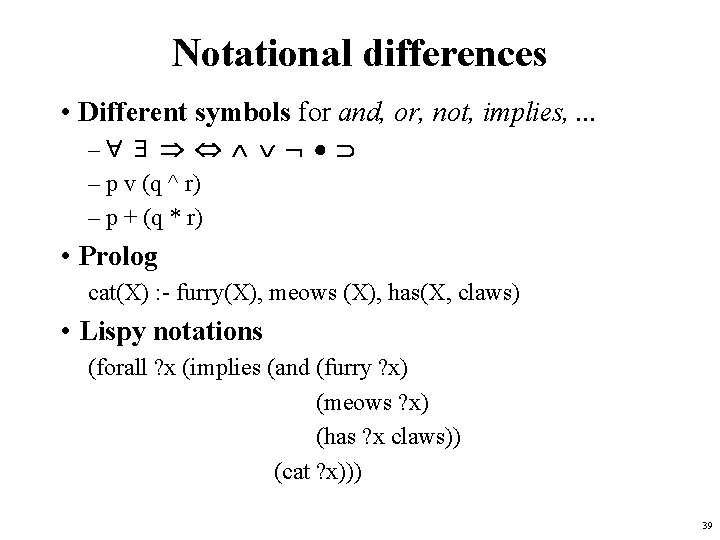Notational differences • Different symbols for and, or, not, implies, . . . – – p v (q ^ r) – p + (q * r) • Prolog cat(X) : - furry(X), meows (X), has(X, claws) • Lispy notations (forall ? x (implies (and (furry ? x) (meows ? x) (has ? x claws)) (cat ? x))) 39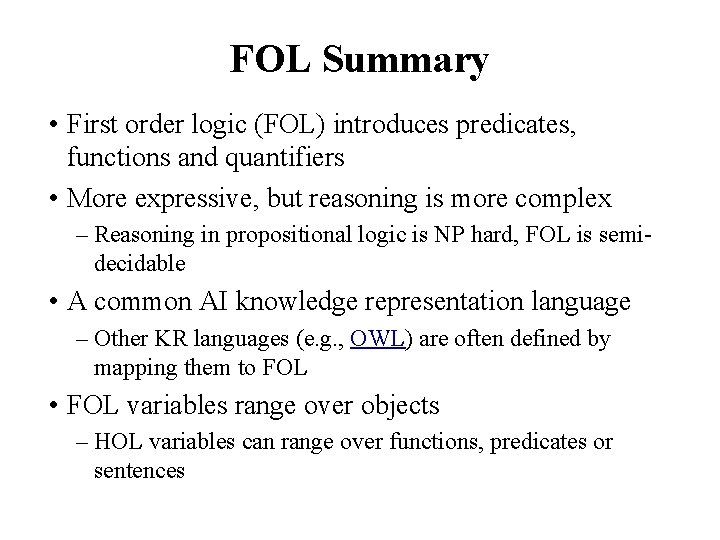FOL Summary • First order logic (FOL) introduces predicates, functions and quantifiers • More expressive, but reasoning is more complex – Reasoning in propositional logic is NP hard, FOL is semidecidable • A common AI knowledge representation language – Other KR languages (e. g. , OWL) are often defined by mapping them to FOL • FOL variables range over objects – HOL variables can range over functions, predicates or sentences